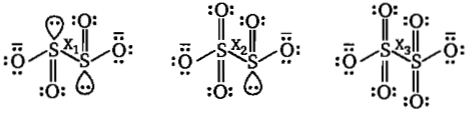# NEET Chemistry The p-Block Elements (XII) Questions Solved

The correct order of $\mathrm{S}—\mathrm{S}$ bond length in the following oxyanion is :

(I) ${\mathrm{S}}_{2}{\mathrm{O}}_{4}^{2-}$                  (II) ${\mathrm{S}}_{2}{\mathrm{O}}_{5}^{2-}$                      (III) ${\mathrm{S}}_{2}{\mathrm{O}}_{6}^{2-}$

(a) I$>$II$>$III                                      (b) I$>$III$>$II

(c) III$>$II$>$I                                       (d) III$>$I$>$II

(a)According to Bents rule ${\mathrm{x}}_{1}>{\mathrm{x}}_{2}>{\mathrm{x}}_{3}$.

Difficulty Level:

• 35%
• 22%
• 35%
• 10%